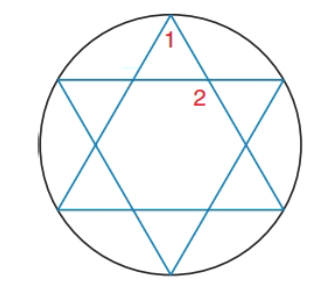Chapter 6.2, Problem 30E### Elementary Geometry for College St...

6th Edition
Daniel C. Alexander + 1 other
ISBN: 9781285195698

#### Solutions

Chapter
Section### Elementary Geometry for College St...

6th Edition
Daniel C. Alexander + 1 other
ISBN: 9781285195698
Textbook Problem
25 views

# For the six-pointed star ( a regular hexagram) inscribed in the circle, find the measures of ∠ 1   and ∠ 2 .To determine

To find:

The measure of 1and2.

Explanation

Given:

Calculation:

If 1 has the vertex on the circle:

mA=m1=mCDE2=mCD+mDE2=2mCD2=mCD=360°6=60°

If 2 has the vertex inside the circle.

### Still sussing out bartleby?

Check out a sample textbook solution.

See a sample solution

#### The Solution to Your Study Problems

Bartleby provides explanations to thousands of textbook problems written by our experts, many with advanced degrees!

Get Started

#### Find more solutions based on key concepts